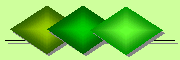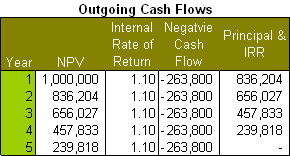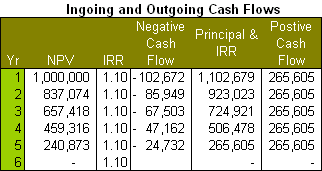De Claron LtdExpress Accountancy services at affordable prices
 Accounting Property Investment Reporting Company Secretarial ServicesHelp

Internal Rate of Return

Internal Rate of Return
IRR is used to measure and compare the profitability of investments. The higher the IRR for an investment project generating incoming cash flows then the more profitable is that investment. Thus the IRR is used as a decision tool to rank investments on the basis of their respective profits. The IRR does this by comparing both incoming and outgoing cash flows of each investment. These cash flows have a discounted rate which reflects their time value and any risk value. The resulting figure is the compounded return rate (i.e. cumulative running total) that, using the IRR, is calculated at a constant annual rate, until both the incoming and outgoing cash flows cancel each other out over time for a nil balance. That is, the Net Present Value (NPV) eventually becomes zero. This is the breakeven point and the higher the IRR then the quicker it takes for this breakeven point to be reached for an investment.

This can be illustrated by looking at outgoing and and outgoing cash flows separately.The above table illustrates a hypothetical investment property which is bought for \$1,000,000 and attracts an interest rate on mortgage repayments. In this example the IRR would equate to the effective interest rate which is 10%. The IRR discounts the cumulative NPV until it become zero. In other words each mortgage repayment reduces the balance unitl the mortgage is repaid which in this case take 5 years. Note that in this case, since only outgoing cash flows are being considered, a lower IRR means that the property attracts less costs and is more desirable than a comparable property with a higher IRR.The above table illustrates an investment property which is already owned and thus has no mortgage. The property is valued at \$1,000,000, split into shops and then leased out. However, in the first two years the property is vacant and thus does not attract rental income. Hence there is no positive cash flows for the period.This risk factor and time value is reflected in the discounted cash flow whereby in the first year the projected value of the property of \$1,100,757 has been discounted to \$1,000,000. The same applies to the second year where the projected value of property of \$1,211,666 has been discounted to \$1,100,757. The cumulative difference in values is the cost of the property to the owner over the original selling price.

In the remaining years, the property does attract income in the form of rent, albeit at staggered levels. This rental income represents the positive cash flow to the investment property and is used to offset the discounted cash flows in the first two years. At the end of the five year period the NPV is again zero. That is it takes five years to break-even on this investment. For properties only generating ingoing cash flows, a higher IRR means greater profitability making the property more desirable as an investment.Now we will consider both ingoing and outgoing cash flows. In the beginning of the first year a property is bought and it attracts an annual mortgage interest rate of 10%. This is the outgoing discounted cash flow. However, the property also produces a rental income of \$265,605 per annum which is the ingoing cash flow. This income is used to pay off the mortgage until at the end of the fifth year the NPV is again nil. That is, it takes five years for the property to pay for itself.

Since the IRR is amortised at a constant rate it can be understood as a summary figure of the profitability of an investment property. As noted this allows comparison with other investment properties by their respective IRR values. This is illustrated by the following example:

There are two prospective investment properties:

• Property A can be obtained with a mortgage that has an annual interest rate of 10% and an administrative fees which brings the effective interest rate to 11% which equates to the IRR.
• Property B can be obtained for a mortgage that has lower annual interest rate of 9% which equates to the IRR.
• The purchase price of both properties is \$1,000,000. If the IRR only represented outgoing cash flows which in both cases is the effective interest rate of mortgage repayments then one would choose the lower IRR. However in the above examples, there are additional ingoing cash flows in terms of rental income. Hence the respective IRRs of both properties do not only represent the respective effective interest rates on their associated mortgages but also the ingoing cash flows from their rental incomes. The IRR is how quickly these ingoing cash flows repay the mortgage for each property. On this basis the more profitable investment is property A even though it has a higher interest rate for its mortgage than property B. This is because property A attracts greater incoming cash flows that pay off its associated mortgage quicker. It takes 3 years to pay off the mortgage for property A instead of 5 years for property B.

IRR Limitations
Despite the usefulness of IRR in comparing the profitability of investments it suffers from the following limitations:

• It is difficult to calculate. As noted the IRR calculates the NPV on the basis of amortising the discounted cash flow over time until the NPV is nil. This process involves multiple iterations for each discounted cash flow over time which in turn maybe sporadic and uneven.
• It make unrealistic assumptions about reinvestment. The IRR assumes that one reinvests in the original investment project or other projects with the same IRR. However, one may decide not to inject money into the original investment or invest in a project with the a lower IRR in the absence of alternatives.
• It can generate multiple values for the same project. This ambuigity arises because ingoing and outgoing cash flows are respectively discounted positively and negatively so their frequency and order can produce different IRR rates.
• It does not consider the costs of capital and hence should not be used to compare projects of different durations
• It does not take inflation into consideration
• Since the Modified Internal Rate of Return (MIRR) address these problems it maybe an alternative method of assessing the profitability of investment projects.